# Two-generator corners group

On the normal 3x3x3 cube, or on the 2x2x2 cube, mix it up using only turns of the Right and Up faces. Then try to solve the corners using only those two faces. You may notice that after you solve the two corners in the bottom face, no more than a simple turn of the U face is necessary to put the other 4 corners into their correct positions. In particular, you never need to swap or 3-cycle the corners. On this page I will prove this curious fact.

As you may have seen on my Rotational Puzzles on Graphs page, in nearly all cases where you have two overlapping faces of a puzzle, at least every even permutation of their pieces is possible. In this case the corners on two adjacent faces form overlapping 4-cycles, so you would expect that all 6!=720 permutations of the corners can be achieved. It turns out that only 5!=120 of them are possible. There are two completely different proofs below.

## First proof

The six corners of two adjacent faces can be combined into 3 pairs of corners. This can be done in many ways, but consider the 5 pairings figured below: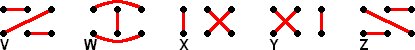This is an interesting set of pairings. With 6 objects, there are 6·5/2 = 15 possible pairs. The 5 patterns above each have 3 pairs, and the 15 possible pairs all occur once. For our purposes, it is important to realise that performing any face twist on any one them will again result in one of the 5 patterns. This already indicates that not every permutation can be achieved - for example swapping two pieces that are not in the same pair results in a pattern different to these five, and so cannot be achieved by turns of the two faces.

The picture below shows the principle. After an F turn, each pairing pattern is turned into some other pairing pattern.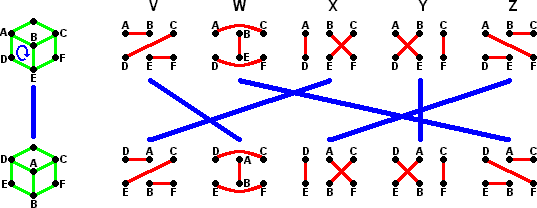Similarly, a turn of the R face changes the patterns around.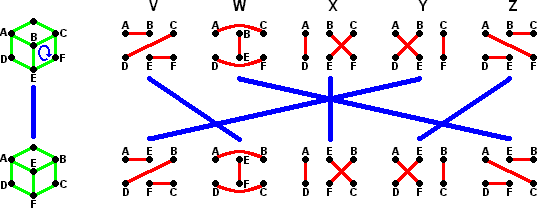The patterns shown above are labelled V-Z. The two possible moves can be considered as permutations of these five patterns. From the picture you can easily see that F corresponds to the permutation (VWZX) and R corresponds to (VWZY). Any corner permutation arrived at by using only F and R turns can thus also be considered a permutation of the 5 patterns V to Z.

The above shows that any achievable permutation of the 6 corners corresponds to a permutation of 5 patterns. There are 5!=120 such permutations. If any two different corner permutations always correspond to different pattern permutations, then (by the pigeonhole principle) there can be no more than 120 possible corner permutations.

So now all that is left is to show that no two distinct corner permutations give the same permutation of the five patterns. Suppose we have two corner permutations, p and q, and that these correspond to the same pattern permutations r. Then pq-1 corresponds to the pattern permutation rr-1, which is the identity. We therefore merely have to show that the only way to mix the corners but still keep all five pairing patterns intact, is by not moving any corner at all.

The 6 corners are labelled as A-F, as shown in the pictures above. Suppose we mix them, but that none of the pairing patterns have changed. Can piece A have moved to position B? Suppose it has. Since pattern V has not changed, piece B must have moved to position A, so that A-B is still a pair in that pattern. In pattern W, pieces A and C are a pair, as are B and E. They must still be a pairs afterwards, and since A and B have swapped, so must C and E. Applying the same reasoning to pattern Y, we find however that D and E should have been swapped instead. This is a contradiction, so A cannot have moved to B. Similar reasoning shows that A cannot have moved to any other place either. It then easily follows that the other pieces cannot have moved either.

The above proof shows that the achievable corner permutations are in exact correspondence with the group of permutations of the five pairing patterns, i.e. that the group is isomorphic to S5.

## Projective geometry proof:

Consider the set of invertible 2x2 matrices. These matrices form a group, because

• the identity matrix is in the set,
• every matrix in the set has an inverse,
• matrix multiplication is associative, and
• multiplying two invertible matrices results in another invertible matrix.

The above is the case for matrices over any number field. Usually the field is R, so all the entries of the matrix are real numbers. In that case this group is infinite - there are infinitely many invertible matrices with real numbers as entries. This group is generally denoted SL2(R).

It is also possible to use a finite field of numbers, for example the integers modulo 5, forming the group SL2(Z5). In this case all the entries of the matrices are numbers in the range 0 to 4, and all the arithmetic is done mod 5. Now there are only finitely many such matrices. Each matrix entry can have at most 5 possibilities, so there at most 54=625 of them. There are in fact somewhat fewer than that because this figure includes non-invertible matrices.

Some matrices are scalar multiples of another, i.e. by multiplying all the entries of one matrix by a single number you get the other matrix. If we consider all such multiples as the same group element then we get another group. We are 'dividing out' a scalar factor to form a quotient group. This group is generally called PGL2(Z5). Let's look at it more closely.

To recap: PGL2(Z5) is the set of invertible 2x2 matrices which have entries which are integers modulo 5, and where scalar multiples of a matrix are considered identical to each other.

Consider the following matrix: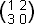Its scalar multiples (modulo 5) are: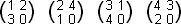Whichever one of these matrices we have, it represents the same element of the group. Therefore, let's always choose the one which has a 1 as the bottom left entry if possible. If there is a 0 at the bottom left, then instead choose the one which has a 1 at the bottom right. In this way we can uniquely choose a representative matrix for any element of the group.

Now that every element of PGL2(Z5) has a uniquely determined representative matrix, we can work out the size of the group by counting those matrices. If the bottom left entry is 1, then there are 5*5 ways of choosing two of the remaining entries of the matrix. When choosing the fourth entry we must ensure the matrix determinant is non-zero (to keep the matrix invertible), so there are only 4 options for that entry. This gives 5*5*4=100 possible matrices. If on the other hand the bottom left entry is zero, the bottom right entry is chosen to be one, and then the two remaining entries have 5*4 possibilities for similar reasons. This gives a total of 100+20=120 matrices. Therefore PGL2(Z5) is a group of size 120.

Vectors are often multiplied by matrices, to effect a rotation or scaling of the vector. Suppose we have a matrix A representing some element of PGL2(Z5), and multiply a vector by it.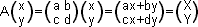The matrix represents the same group element regardless of scalar multiples, so the length of the vectors we get has to be ignored. The only important part is the direction of the vector. Therefore we can just scale the vectors just like we did the matrix, to get a particular representative vector for a direction. This means that if the bottom coordinate is non-zero we can scale so that it becomes 1, and if the bottom coordinate is 0 then we scale the vector so that the top coordinate is 1. This leaves us only 6 vectors that we are working with: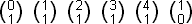We can denote these by the value of x/y, namely 0, 1, 2, 3, 4, and inf.

Multiplying one of these 6 vectors by a matrix from PGL2(Z5) results again in one of these six vectors. As the matrix is invertible, this mapping is one-to-one. Therefore, such a matrix permutes these 6 vectors, and can be thought of as an element in S6.

Let's see what the following matrices do to the six vectors: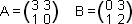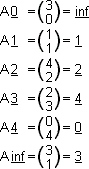So the matrix A cycles (3 4 0 inf). You can check in the same way that B cycles (1 2 inf 0).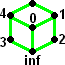Now we have at last arrived at a point where we can connect this topic with the Rubik's Cube. Let's label the 6 corners of the cube that lie in the F or R faces as shown in the picture. Clearly turns of the faces correspond to the elements A and B in the group PGL2(Z5). Therefore, the group generated by the two face turns corresponds to a subgroup of PGL2(Z5). We already saw that PGL2(Z5) has 120 elements, which means that there are no more than 120 possible corner positions that can be achieved. With a little work you can show that you can actually achieve 120 different permutations, so this proves that the corner permutation group is isomorphic to PGL2(Z5) itself.

Combining this proof with the previous one, we find that PGL2(Z5) must be isomorphic to S5. The only difference between these two representations of the same group is that the first naturally acts as a permutation group of 6 items (the corner pieces) whereas the second acts naturally as a permutation group of 5 items (the pairing patterns).